Contents >> Applied Mathematics >> Matrix Algebra >> Principles of Matrix Calculation >> Example

 Matrix Algebra - Example Example Solve the system of linear equations, using the matrix methods: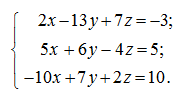S o l u t i o n Let's record the given system of linear equations in the matrix form: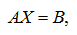where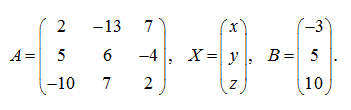The solution of the given system of linear equations in the matrix form looks like: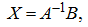where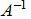– an inverse matrix to a matrix A . The determinant of the matrix A of coefficients is equal: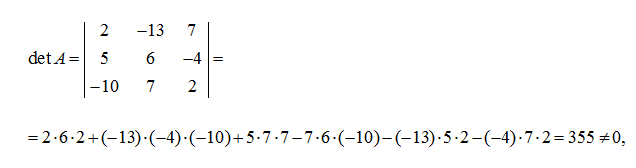consequently, the matrix A has an inverse matrix. First we’ll find an adjoint matrix Ã which in the given example looks like: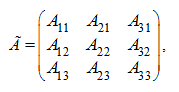where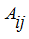– algebraic additions of appropriating elements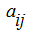of matrix A . In our case we’ll receive: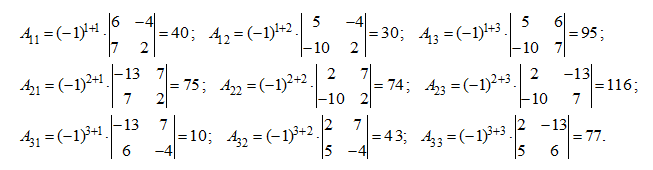Thus,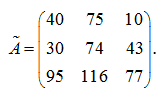Then the inverse matrixis equal: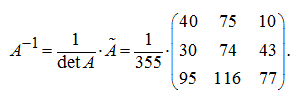Now we’ll find the solution of the given system of equations. As,then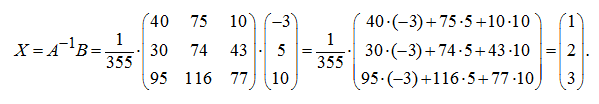Thus, the solution of the given system of equations: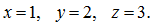< Previous Contents Next >